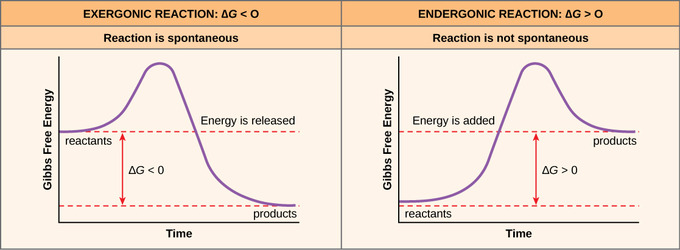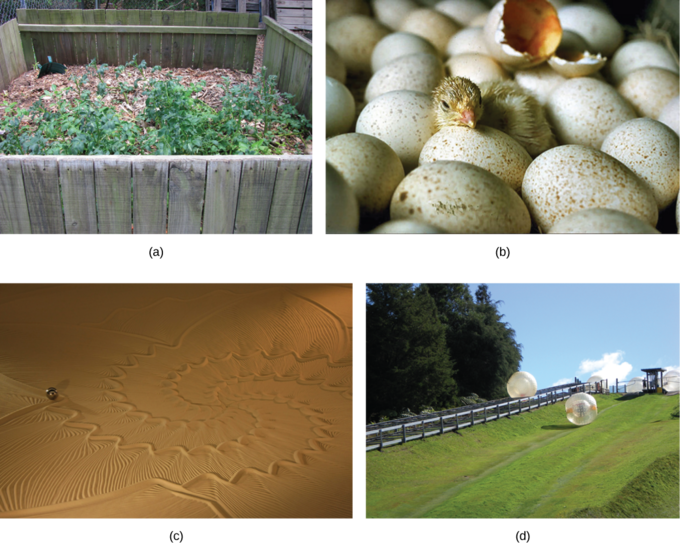# 6.2A: Free Energy

•• Contributed by Boundless
• General Microbiology at Boundless

Free energy, called Gibbs free energy (G), is usable energy or energy that is available to do work.

Learning Objectives

• Discuss the concept of free energy.

## Key Points

• Every chemical reaction involves a change in free energy, called delta G (∆G).
• To calculate ∆G, subtract the amount of energy lost to entropy (∆S) from the total energy change of the system; this total energy change in the system is called enthalpy (∆H ): ΔG=ΔH−TΔS.
• Endergonic reactions require an input of energy; the ∆G for that reaction will be a positive value.
• Exergonic reactions release free energy; the ∆G for that reaction will be a negative value.

## Key Terms

• exergonic reaction: A chemical reaction where the change in the Gibbs free energy is negative, indicating a spontaneous reaction
• endergonic reaction: A chemical reaction in which the standard change in free energy is positive, and energy is absorbed
• Gibbs free energy: The difference between the enthalpy of a system and the product of its entropy and absolute temperature

## Free Energy

Since chemical reactions release energy when energy-storing bonds are broken, how is the energy associated with chemical reactions quantified and expressed? How can the energy released from one reaction be compared to that of another reaction?

A measurement of free energy is used to quantitate these energy transfers. Free energy is called Gibbs free energy (G) after Josiah Willard Gibbs, the scientist who developed the measurement. Recall that according to the second law of thermodynamics, all energy transfers involve the loss of some amount of energy in an unusable form such as heat, resulting in entropy. Gibbs free energy specifically refers to the energy associated with a chemical reaction that is available after accounting for entropy. In other words, Gibbs free energy is usable energy or energy that is available to do work.

## Calculating ∆G

Every chemical reaction involves a change in free energy, called delta G (∆G). The change in free energy can be calculated for any system that undergoes a change, such as a chemical reaction. To calculate ∆G, subtract the amount of energy lost to entropy (denoted as ∆S) from the total energy change of the system. This total energy change in the system is called enthalpy and is denoted as ∆H. The formula for calculating ∆G is as follows, where the symbol T refers to absolute temperature in Kelvin (degrees Celsius + 273): G=ΔH−TΔS.

The standard free energy change of a chemical reaction is expressed as an amount of energy per mole of the reaction product (either in kilojoules or kilocalories, kJ/mol or kcal/mol; 1 kJ = 0.239 kcal) under standard pH, temperature, and pressure conditions. Standard pH, temperature, and pressure conditions are generally calculated at pH 7.0 in biological systems, 25 degrees Celsius, and 100 kilopascals (1 atm pressure), respectively. It is important to note that cellular conditions vary considerably from these standard conditions; therefore, standard calculated ∆G values for biological reactions will be different inside the cell.

## Endergonic and Exergonic Reactions

If energy is released during a chemical reaction, then the resulting value from the above equation will be a negative number. In other words, reactions that release energy have a ∆G < 0. A negative ∆G also means that the products of the reaction have less free energy than the reactants because they gave off some free energy during the reaction. Reactions that have a negative ∆G and, consequently, release free energy, are called exergonic reactions. Exergonic means energy is exiting the system. These reactions are also referred to as spontaneous reactions because they can occur without the addition of energy into the system. Understanding which chemical reactions are spontaneous and release free energy is extremely useful for biologists because these reactions can be harnessed to perform work inside the cell. An important distinction must be drawn between the term spontaneous and the idea of a chemical reaction that occurs immediately. Contrary to the everyday use of the term, a spontaneous reaction is not one that suddenly or quickly occurs. The rusting of iron is an example of a spontaneous reaction that occurs slowly, little by little, over time.

If a chemical reaction requires an input of energy rather than releasing energy, then the ∆G for that reaction will be a positive value. In this case, the products have more free energy than the reactants. Thus, the products of these reactions can be thought of as energy-storing molecules. These chemical reactions are called endergonic reactions; they are non-spontaneous. An endergonic reaction will not take place on its own without the addition of free energy.Figure $$\PageIndex{1}$$: Exergonic and Endergonic Reactions: Exergonic and endergonic reactions result in changes in Gibbs free energy. Exergonic reactions release energy; endergonic reactions require energy to proceed.

## Free Energy and Biological Processes

In a living cell, chemical reactions are constantly moving towards equilibrium, but never reach it. A living cell is an open system: materials pass in and out, the cell recycles the products of certain chemical reactions into other reactions, and chemical equilibrium is never reached. In this way, living organisms are in a constant energy-requiring, uphill battle against equilibrium and entropy.

When complex molecules, such as starches, are built from simpler molecules, such as sugars, the anabolic process requires energy. Therefore, the chemical reactions involved in anabolic processes are endergonic reactions. On the other hand, the catabolic process of breaking sugar down into simpler molecules releases energy in a series of exergonic reactions. As in the example of rust above, the breakdown of sugar involves spontaneous reactions, but these reactions don’t occur instantaneously. An important concept in the study of metabolism and energy is that of chemical equilibrium. Most chemical reactions are reversible. They can proceed in both directions, releasing energy into their environment in one direction, and absorbing it from the environment in the other direction.Figure $$\PageIndex{1}$$: Endergonic and Exergonic Processes: Shown are some examples of endergonic processes (ones that require energy) and exergonic processes (ones that release energy). These include (a) a compost pile decomposing, (b) a chick hatching from a fertilized egg, (c) sand art being destroyed, and (d) a ball rolling down a hill.